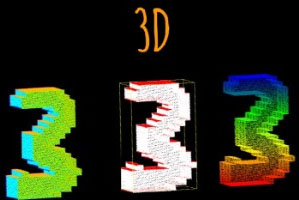# 3D MNIST 数字识别数据★★★☆☆数据采集定制数据标注定制

# 内容

## full_dataset_vectors.h5

• X_train（10000,4096）
• y_train（10000）
• X_test（2000,4096）
• y_test（2000）

``` with h5py.File("../input/train_point_clouds.h5", "r") as hf:
X_train = hf["X_train"][:]
y_train = hf["y_train"][:]
X_test = hf["X_test"][:]
y_test = hf["y_test"][:]
本文来自可思数据(sykv.com)，转载请联系本站及注明出处 ```

## train_point_clouds.h5＆test_point_clouds.h5

5000（火车）和1000（测试）3D点云以HDF5文件格式存储。点云的平均值为零，最大维度范围为1。

• “点”数据集：x, y, z点云中每个3D点的坐标。
• “法线”数据集：nx, ny, nz单位正常的组件与每个点相关联。
• “img”数据集：原始的mnist图像。
• “label”属性：原始的mnist标签。

```with h5py.File("../input/train_point_clouds.h5", "r") as hf:
a = hf["0"]
b = hf["1"]
digit_a = (a["img"][:], a["points"][:], a.attrs["label"])
digit_b = (b["img"][:], b["points"][:], b.attrs["label"])
可思数据-人工智能资讯平台sykv.com ```

## plot3D.py

• array_to_color 将1D数组转换为rgb值，color在plot_points（）中用作kwarg 可思数据sykv.com,sykv.cn

• plot_points(xyz, colors=None, size=0.1, axis=False)

可思数据-AI,sykv.com人工智能,深度学习,机器学习,神经网络

• plot_voxelgrid(v_grid, cmap="Oranges", axis=False)

可思数据-AI,sykv.com智能驾驶,人脸识别,区块链,大数据

¤ 此数据集库仅供研究和教育用途，不得用于任何商业用途。
¤ 如果您在任何出版物或报告中使用该数据库，则必须添加引用来源。
¤ 版权归作者及所在组织所有。
¤ 如用于商业用途，请联系版权所有人。
¤ 本站数据来源于网络如有侵权请联系我们。• ##### 客服QQ